# Program to check Pronic Number in Java

In this tutorial you will learn about the Program to check Pronic Number in Java and its application with practical example.

In this tutorial, we will learn to create a Java Program to check Pronic Numbers in Java using Java programming.

## Prerequisites

Before starting with this tutorial we assume that you are best aware of the following Java programming topics:

• Java Operators.
• Basic Input and Output function in Java.
• Class and Object in Java.
• Basic Java programming.
• If-else statements in Java.
• For loop in Java.

## What is Pronic or Heteromecic  number?

A number is called a Pronic or Heterimecic if product or two consecutive number giver the number itself.

Example :

1) 42 => 6 * 7 => gives 42 6 and 7 are  consecutive  number.

2) 50 => 10 * 5 =: gives 50 but 5 and 10 are not consecutive  number.

## Program to check Pronic Number in Java

In this our program we will find given number is a Pronic number or not in using java programming. We would first declared and initialized the required variables. Next, we would prompt user to input the a number.Later we  find number is Pronic number or not let’s have a look at the code.

## Output

Pronic Number.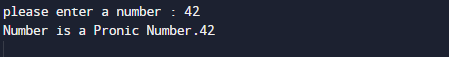Not a Pronic Number.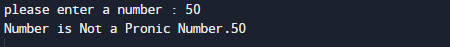In the above program, we have first declared and initialized a set variables required in the program.

• number= it will hold entered number.
• result= it will hold result.
• i= i for iteration .

After declaring variables in the next statement user will be prompted to enter a value and  which will be assigned to variable ‘number’.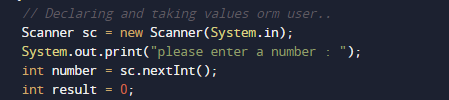And after that we calculate the product of two consecutive number with in the number gives the number itself then the number is a pronic.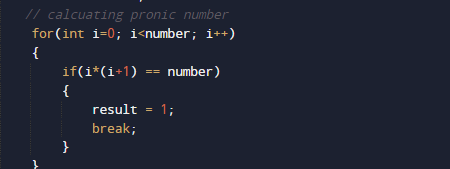So the given number called Pronic if “sum of its two consecutive number gives the number” Called Pronic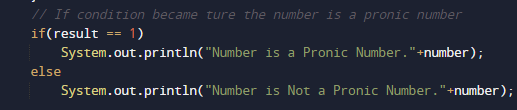If not then the number is not a Pronic.

In this tutorial we have learn about the Program to check Pronic Number in Java and its application with practical example. I hope you will like this tutorial.